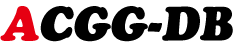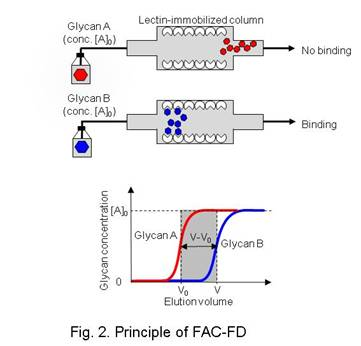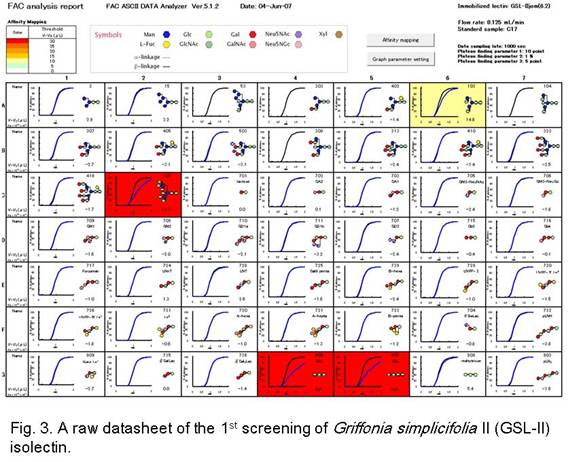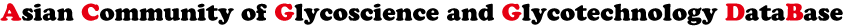# Lectin Frontier DataBaseProtocols of FAC-FDPrinciple of FAC-FD

The analytical principle is literally based on quantitative affinity chromatography. An excess volume of glycan-containing solution is applied onto the lectin-immobilized column. If the glycan binds to the immobilized lectin, the elution front volume (V) is delayed compared to the negative control glycan (V0) (Fig. 2).

The V-V0 value corresponds to the binding affinity between the glycan and the immobilized lectin. From the obtained V-V0 and Bt (the effective lectin content expressed in mol), Kd is calculated from the following formula:

[A]0 (V-V0) = Bt [A]0 / ([A]0 + Kd) (equation 1)

,where [A]0 is the initial glycan concentration in M. Since [A]0 (~5.0 x 10-9 M) required for FAC-FD analysis is negligibly small compared with the Kd (10-3-10-7 M) of general sugar-protein interactions, the following formula can be derived from equation 1:

Kd = Bt / (V-V0), if Kd >>[A]0 (equation 2)

,where the affinity constant (Ka) is the inverse of Kd (Ka =1/ Kd).

Analytical scheme

FAC-FD analysis is performed using the automated FAC-FD system in a highly systematic manner. The analytical scheme is divided into four steps: (i) preparation of lectin-immobilized columns, (ii) 1st screening, (iii) 2nd screening, and (iv) concentration-dependence analysis.

Preparation of lectin-immobilized columns: In most cases, lectins are immobilized on N-hydroxysuccinimide (NHS)-activated Sepharose 4FF using amine coupling chemistry.1st screening using 49 selected PA-glycans: To validate the prepared lectin column, binding to 49 PA-glycans, representing glycan structures derived from various glycoconjugates, is examined as the first screening. If little or no binding is observed for the first 49 glycans, another column, immobilizing a still higher concentration of the same lectin, is prepared. An example of GSL-II isolectin is shown in Fig. 3.

2nd screening using a full panel of PA-glycans: If sufficient binding is observed for a specific group of glycan structures, the second screening using a full panel of PA-glycans for more detailed and precise analysis is carried out. In particular cases, the third step of extended analysis is optionally performed using non-standard or specially elaborated glycans.

Concentration-dependence analysis: Finally, the effective ligand content (Bt) value of the immobilized lectin is determined by a so-called concentration-dependence analysis followed by a Woolf-Hofstee-type plot for determination of Kd as well as Bt using an appropriate positive saccharide derivative. In this DB, data are shown in an actual measurement (V-V0) as well as an association constant (Ka).

LfDB Lectin Frontier DataBase